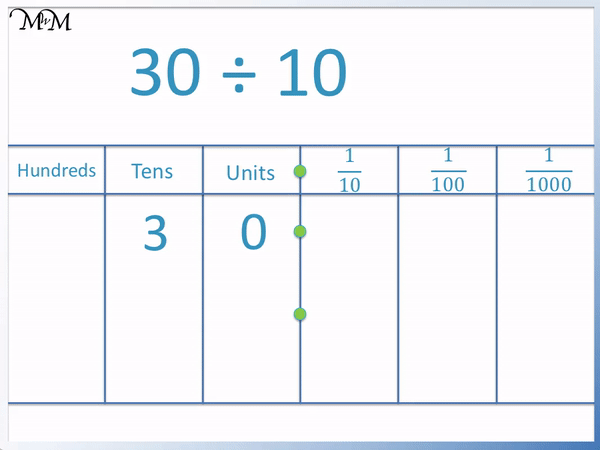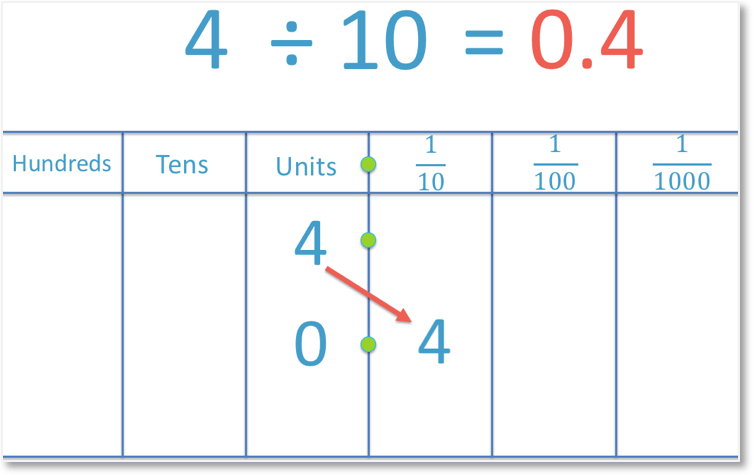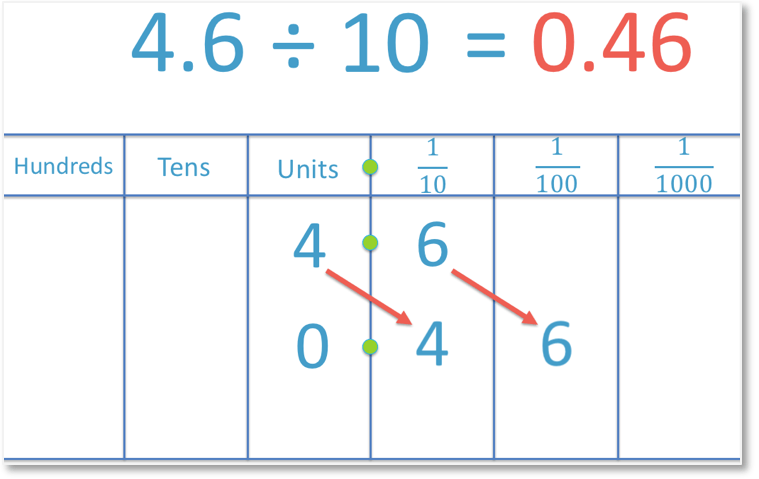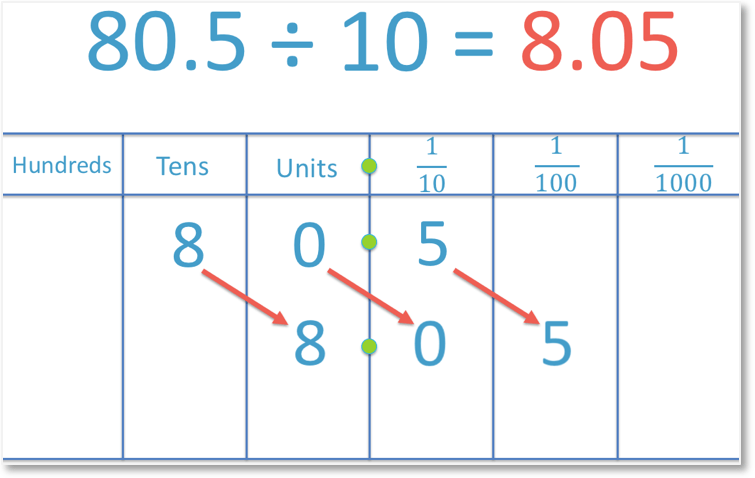# Dividing Decimals by 10

Dividing Decimals by 10• To divide a number by ten, move each digit in that number one place to the right.
• We will look at the example of dividing 30 by 10.
• ‘3’ moves from the tens column into the units column.
• The ‘0’ moves from the units column past the decimal point into the tenths column.
• 30 ÷ 10 = 3.0
• If the only digit after the decimal point is a zero, we do not write it.
• 30 ÷ 10 = 3
To divide a number by 10, move all of its digits one places to the right.

Dividing by 10 has the effect of removing a zero digit from a number that has a ‘0’ in its units column.• To divide a number by 10, all digits of this number move one place value column to the right.
• In this example we will divide the number 91 by 10.
• The digit ‘9’ moves from the tens column to the units column.
• The digit ‘1’ moves from the units column to the tenths column.
• 91 ÷ 10 = 9.1Supporting Lessons# How to Divide a Decimal Number by 10

To divide a number by 10 we move each digit in that number one place value column to the right.

If a number has a zero in its units column, then dividing this number by ten has the effect of removing this zero.In the example above, each digit in the number 30 has been moved one place to the right. So, 30 becomes 3.0.

If the only digit after a decimal point of a number are zero then there is no need to write these zeros or the decimal point.

Instead of writing ‘3.0’, it is better to write ‘3’.

We also know from our times tables that 30 ÷ 10 = 3.

The number 30 is an example of a number that has a ‘0’ in its units column (or a number that ends in a zero).

We can see that if the number ends in a zero then dividing it by ten has the same effect as removing this zero digit.

30 became 3.

This is a trick that will only work for numbers with a zero in the units column.

It is important to understand how dividing by 10 works because not all numbers that we divide by 10 will end in a zero in their units column.

For example:To divide 4 by 10, we move the 4 one place to the right. So, it moves from the units column into the tenths column. Because there is no longer a digit in the units column, we write in a zero.

Therefore 4 ÷ 10 = 0.4.

If there are no digits before the decimal point of a number, one zero digit it always written in the units column to show this.

It is important to make sure that when we are dividing a number with more than one significant digit that we move every digit one place to the right.

For example:To divide 91 by 10, we move both digits one place to the right. We can move them one at a time to make sure that we don’t make any mistakes.

We start by moving the 9 from the tens column into the units column. The ‘1’ will then follow, remaining to the right of the ‘9’.

We move the ‘1’ from the units column into the tenths column. Therefore 91 ÷ 10 = 9.1.

We can see that the digits of ‘9’ and ‘1’ remain in this order, with the ‘9’ directly to the left of the ‘1’.

Now we will divide a decimal number by 10. For example:In this example we still follow the same place value rules. Each digit in 4.6 must move one place to the right. We start by moving the 4 from the units column into the tenths column and then the 6 will follow.

The 6 is moved from the tenths column into the hundredths column.

4.6 divided by 10 is 0.46

Remember that because there are no digits in the units column or anywhere before the decimal point, we must write a single zero digit in the units column to show this.

We follow the same rules when dividing a decimal number that is less than 1 by 10.

For example:We begin by moving the 7 one place to the right, from the tenths column into the hundredths column. The 2 will then follow. It moves from the hundredths column into the thousandths column.

As there are no digits in either the units column or the tenths column, we must make sure that we write zeros in here to show that their values are zero.

Alternatively, we can move the zero from the units column into the tenths column.

And so, 0.72 ÷ 10 = 0.072.

We always leave a single zero digit in the units column before the decimal point for numbers that have no more digits remaining before the decimal point.

We also put zeros between the decimal point and the first non-zero decimal digit.

We need to be more careful when dealing with numbers where there is a zero between two other digits.

For example:We must make sure that we move every digit in 80.5 one place to the right, including the zero.

We move the 8 from the tens column into the units column, we move the 0 from the units column into the tenths column and we move the 5 from the tenths column into the hundredths column.

And so, 80.5 ÷ 10 = 8.05.

We can see that there are three digits ‘8’, ‘0’ and ‘5’ in this order in the original question.

We can see that these three digits remain in this order, immediately next to each other in the answer as well.

When teaching dividing by ten, it is worth making sure that the answer is sensible by comparing it to an easier example.

In this example we know that 80 divided by 10 is 8 and so we know that when we divide 80.5 by 10, we will have an 8 in the units column as well.

The digits of ‘0’ and ‘5’ will then follow the ‘8’.Now try our lesson on Dividing by 100 where we learn how to divide a number by 100.error: Content is protected !!